# 八大排序算法的Python实现

+关注继续查看1、插入排序

1. def insert_sort(lists):
2.     # 插入排序
3.     count = len(lists)
4.     for i in range(1, count):
5.         key = lists[i]
6.         j = i - 1
7.         while j >= 0
8.             if lists[j] > key:
9.                 lists[j + 1] = lists[j]
10.                 lists[j] = key
11.             j -= 1
12.     return lists

2、希尔排序

1. def shell_sort(lists):
2.     # 希尔排序
3.     count = len(lists)
4.     step = 2
5.     group = count / step
6.     while group > 0
7.         for i in range(0, group):
8.             j = i + group
9.             while j < count:
10.                 k = j - group
11.                 key = lists[j]
12.                 while k >= 0
13.                     if lists[k] > key:
14.                         lists[k + group] = lists[k]
15.                         lists[k] = key
16.                     k -= group
17.                 j += group
18.         group /= step
19.     return lists

3、冒泡排序

1. def bubble_sort(lists):
2.     # 冒泡排序
3.     count = len(lists)
4.     for i in range(0, count):
5.         for j in range(i + 1, count):
6.             if lists[i] > lists[j]:
7.                 lists[i], lists[j] = lists[j], lists[i]
8.     return lists

4、快速排序

1. def quick_sort(lists, left, right):
2.     # 快速排序
3.     if left >= right:
4.         return lists
5.     key = lists[left]
6.     low = left
7.     high = right
8.     while left < right:
9.         while left < right and lists[right] >= key:
10.             right -= 1
11.         lists[left] = lists[right]
12.         while left < right and lists[left] <= key:
13.             left += 1
14.         lists[right] = lists[left]
15.     lists[right] = key
16.     quick_sort(lists, low, left - 1
17.     quick_sort(lists, left + 1, high)
18.     return lists

5、直接选择排序

1. def select_sort(lists):
2.     # 选择排序
3.     count = len(lists)
4.     for i in range(0, count):
5.         min = i
6.         for j in range(i + 1, count):
7.             if lists[min] > lists[j]:
8.                 min = j
9.         lists[min], lists[i] = lists[i], lists[min]
10.     return lists

6、堆排序

1. # 调整堆
3.     lchild = 2 * i + 1
4.     rchild = 2 * i + 2
5.     max = i
6.     if i < size / 2
7.         if lchild < size and lists[lchild] > lists[max]:
8.             max = lchild
9.         if rchild < size and lists[rchild] > lists[max]:
10.             max = rchild
11.         if max != i:
12.             lists[max], lists[i] = lists[i], lists[max]
14.
15. # 创建堆
16. def build_heap(lists, size):
17.     for i in range(0, (size/2))[::-1]:
19.
20. # 堆排序
21. def heap_sort(lists):
22.     size = len(lists)
23.     build_heap(lists, size)
24.     for i in range(0, size)[::-1]:
25.         lists, lists[i] = lists[i], lists[0

7、归并排序

1. def merge(left, right):
2.     i, j = 00
3.     result = []
4.     while i < len(left) and j < len(right):
5.         if left[i] <= right[j]:
6.             result.append(left[i])
7.             i += 1
8.         else
9.             result.append(right[j])
10.             j += 1
11.     result += left[i:]
12.     result += right[j:]
13.     return result
14.
15. def merge_sort(lists):
16.     # 归并排序
17.     if len(lists) <= 1
18.         return lists
19.     num = len(lists) / 2
20.     left = merge_sort(lists[:num])
21.     right = merge_sort(lists[num:])
22.     return merge(left, right)

8、基数排序

1. import math
4.     bucket = [[] for i in range(radix)]
5.     for i in range(1, k+1):
6.         for j in lists:
8.         del lists[:]
9.         for z in bucket:
10.             lists += z
11.             del z[:]
12.     return listsPython实现一个简单的区块链

217 0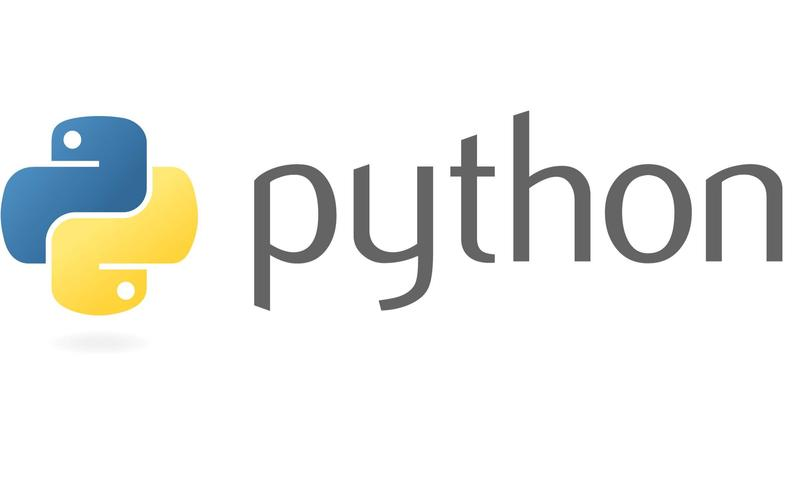python 实现pacs功能 推送下拉影像
python 实现dcmtk关联pacs功能 推送下拉影像
123 0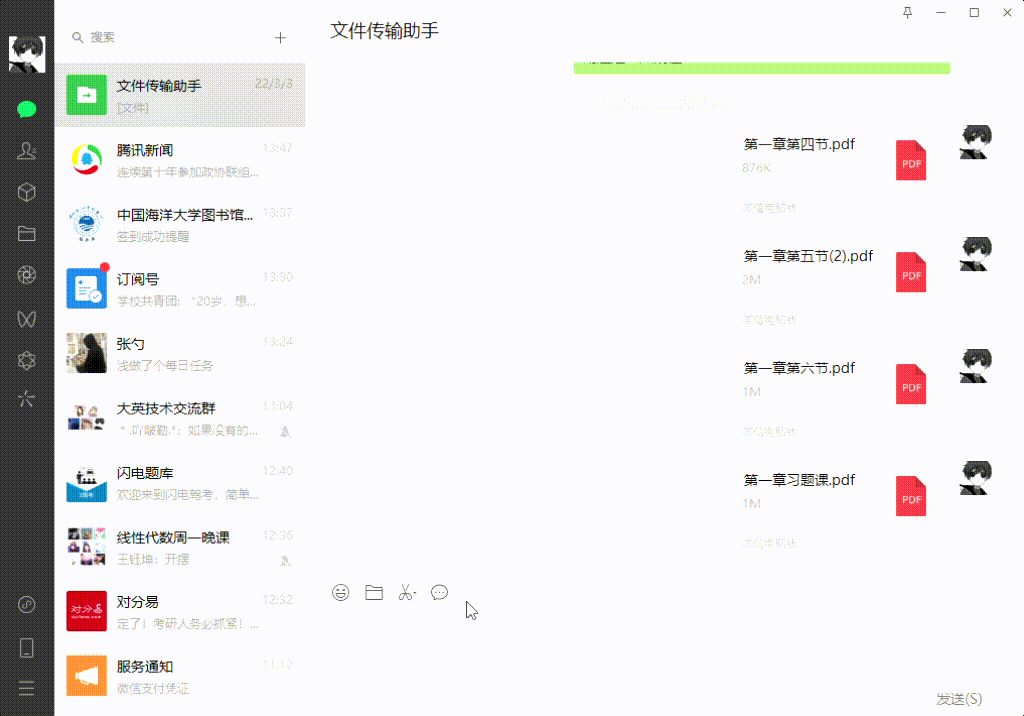Python实现微信消息连续发送
Python实现微信消息连续发送
190 0Python print() 打印两个 list ，实现中间换行
Python print() 打印两个 list ，实现中间换行
332 0Python分分钟实现图书管理系统(含代码)
Python分分钟实现图书管理系统(含代码)
129 0Python：使用PyJWT实现JSON Web Tokens加密解密
Python：使用PyJWT实现JSON Web Tokens加密解密
135 0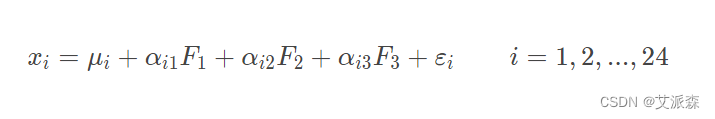Python实现因子分析（附案例实战）
Python实现因子分析（附案例实战）
297 0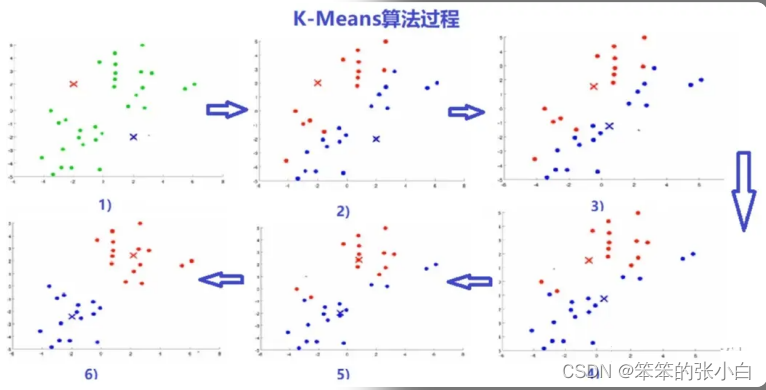Python实现聚类分析和数据降维
Python实现聚类分析和数据降维
451 0Python实现线性回归和梯度下降算法
Python实现线性回归和梯度下降算法
88 0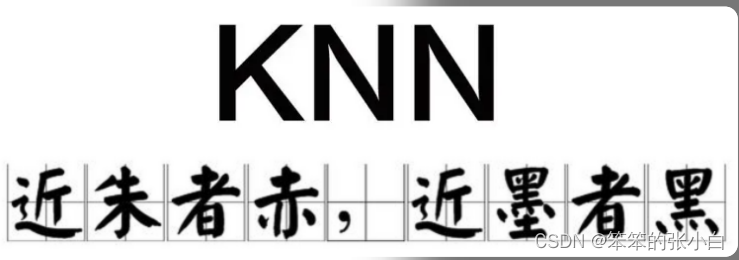Python实现KNN算法和交叉验证
Python实现KNN算法和交叉验证
154 0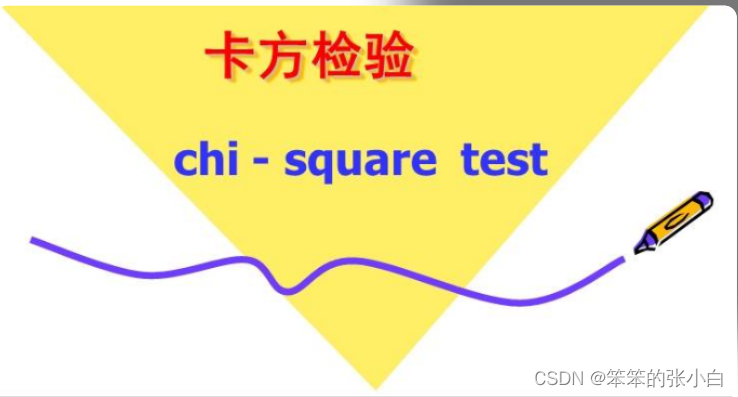Python实现卡方检验和相关性分析
Python实现卡方检验和相关性分析
437 0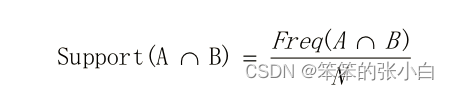162 0

Python基础快速入门实战教程378048